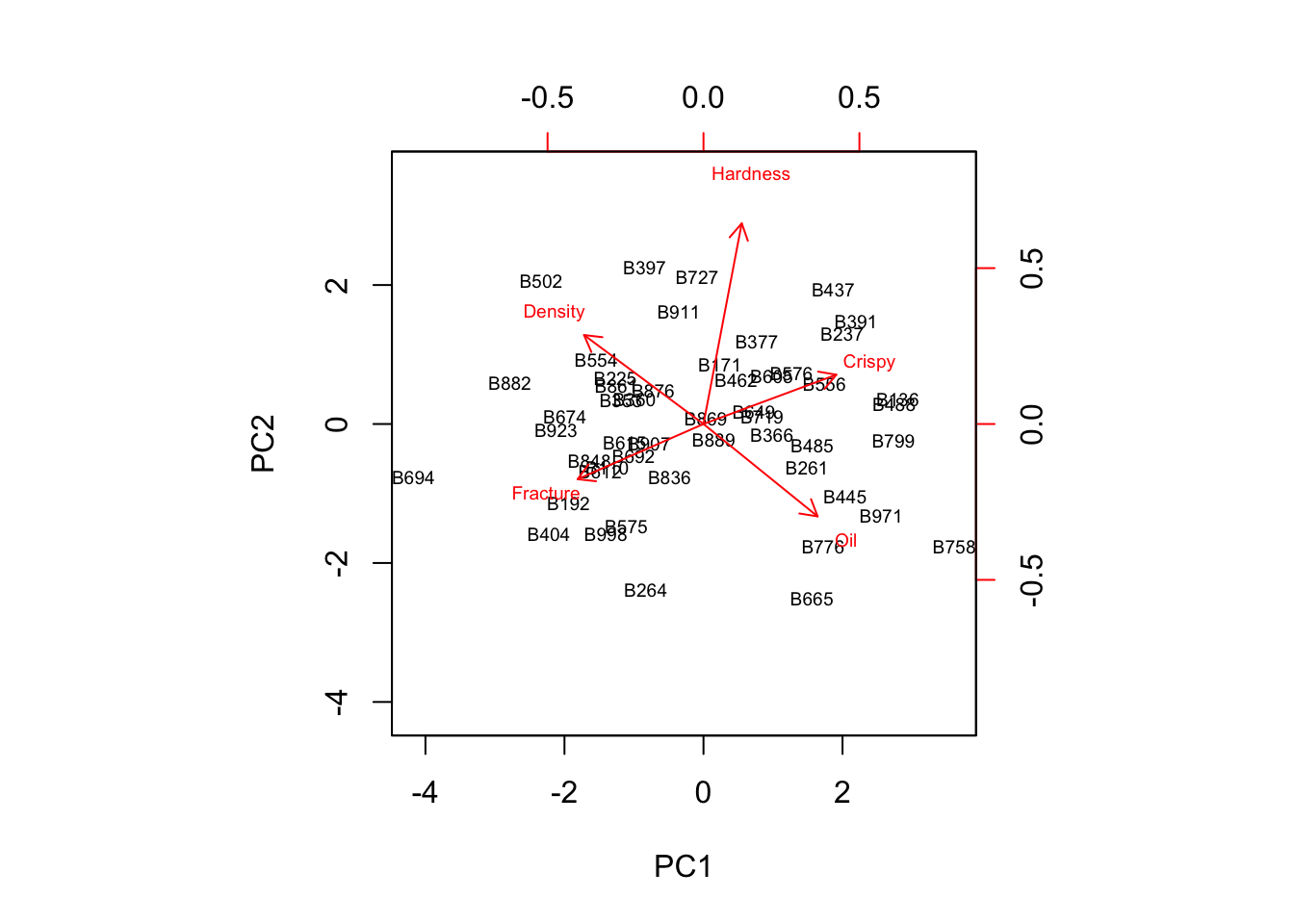In the previous sections we learned about PCA. We worked out an example from scratch to emphasis the mechanics behind PCA. In general, however, we rely on one of the implementations built-in in the R stats package to conduct a PCA. In R there are two main implementations for PCA; prcomp() and princomp(). Both functions implement PCA, however the princomp() function uses the spectral decomposition approach, whereas the the prcomp() function uses singular value decomposition (SVD). According to the R help, SVD has slightly better numerical accuracy.

In this section we revisit the food-texture data set and briefly showcase PCA by applying the R machinery and in particular the prcomp() function.

#### The data

food <- read.csv("https://userpage.fu-berlin.de/soga/300/30100_data_sets/food-texture.csv")
rownames(food) <- food$X food <- food[, 2:ncol(food)] # exclude first column from data set str(food) ## 'data.frame': 50 obs. of 5 variables: ##$ Oil     : num  16.5 17.7 16.2 16.7 16.3 19.1 18.4 17.5 15.7 16.4 ...
##  $Density : int 2955 2660 2870 2920 2975 2790 2750 2770 2955 2945 ... ##$ Crispy  : int  10 14 12 10 11 13 13 10 11 11 ...
##  $Fracture: int 23 9 17 31 26 16 17 26 23 24 ... ##$ Hardness: int  97 139 143 95 143 189 114 63 123 132 ...

The data set consists of 50 rows (observations) and 5 columns (features). By calculating the mean and the standard deviation of the features we realize that the variables are not on the same scale. Hence, we center and scale the variables to have a mean of zero and standard deviation of one.

apply(food, 2, mean)
##      Oil  Density   Crispy Fracture Hardness
##   17.202 2857.600   11.520   20.860  128.180
apply(food, 2, sd)
##        Oil    Density     Crispy   Fracture   Hardness
##   1.592007 124.499980   1.775571   5.466073  31.127578

#### The prcomp object

In order to center and standardize the variables we add center = TRUE and scale = TRUE to the function call prcomp().

food.pca = prcomp(food, center = TRUE, scale = TRUE)

The output from prcomp(), a prcomp object, contains a number of useful quantities.

names(food.pca)
##  "sdev"     "rotation" "center"   "scale"    "x"

The prcomp object stores the eigenvectors as a matrix in the rotation attribute. The name relates to the term rotation matrix and emphasis that a matrix-multiplication of the data-matrix with the rotation matrix returns the coordinates of the data in the rotated coordinate system, and thus the principal component scores.

food.pca$rotation ## PC1 PC2 PC3 PC4 PC5 ## Oil 0.4575334 -0.3704389 0.65903020 -0.4679449 0.01204121 ## Density -0.4787455 0.3567500 0.01623973 -0.7184632 0.35648161 ## Crispy 0.5323877 0.1976610 -0.17888443 0.1325269 0.79242064 ## Fracture -0.5044769 -0.2212399 0.54227938 0.4569317 0.44011646 ## Hardness 0.1534026 0.8046661 0.48923298 0.1961843 -0.22614798 The sdev attribute of the prcomp object contains the standard deviation of each principal component as a measure of variance. food.pca$sdev
##  1.7410380 1.1382907 0.5568207 0.4918537 0.3480110

In order to get the variance explained by each principal component we square the standard deviation.

food.pca.var = food.pca$sdev^2 food.pca.var ##  3.0312132 1.2957058 0.3100493 0.2419201 0.1211116 To compute the proportion of variance explained by each principal component, we simply divide the variance explained by each principal component by the total variance explained by all principal components: food.pca.ve <- food.pca.var/sum(food.pca.var) food.pca.ve ##  0.60624263 0.25914115 0.06200987 0.04838402 0.02422233 We see that the first principal component explains 61% of the variance in the data, the second principal component explains 26% of the variance, and so forth. Finally, we are interested in the principal component scores, which correspond to the projection of the original data on the directions of the principal component. The principal component scores are stored in x attribute of the prcomp object. We take a look at the first 10 entries of food.pca$x by applying the head() function.

# Principal component scores
head(food.pca\$x, 10)
##             PC1        PC2        PC3         PC4          PC5
## B110 -1.3832112 -0.6194061 -0.4025115 -0.48680785 -0.005948156
## B136  2.7944769  0.3537246 -1.0760269  0.25580128 -0.488773246
## B171  0.2375564  0.8614582 -0.6115486  0.02952179 -0.176323309
## B192 -1.9393406 -1.1417366  0.4379471  0.31253089  0.554021980
## B225 -1.2679371  0.6634646  0.4371652  0.07190367  0.403450293
## B237  1.9974564  1.2983543  1.1015333 -0.08025960 -0.351879906
## B261  1.4881878 -0.6326525 -0.2730264 -0.03277424  0.153700961
## B264 -0.8288636 -2.3825485 -0.2494352  0.32334864 -0.039526092
## B353 -1.1851533  0.3501810 -0.4257859 -0.01315433  0.245396437
## B360 -0.9934626  0.3508258  0.1033445 -0.02088279  0.237188926

The columns of the matrix correspond to principal component score vectors. That is, the kth column is the kth principal component score vector.

#### Visualization and interpretation

The biplot is a very popular way for visualization of results from PCA, as it combines both, the principal component scores and the loading vectors in a single biplot display. In R we simply call the biplot() function. The scale = 0 argument to biplot() ensures that the arrows are scaled to represent the loadings.

biplot(food.pca, scale = 0, cex = 0.6)In the biplot the observations are labeled by the observation number (e.g. the row name in data frame). The position in the plot represents the scores for the first two principal components. The original variables are shown as vectors (arrows). They begin at the origin $$[0,0]$$ and extend to coordinates given by the first two principal component loading vectors. For example, the loading for Oil on the first component is 0.46, and its loading on the second principal component -0.48 (the label “Oil” is centered at the point (0.46, -0.48)).